# GEOMETRIC PROGRESSION - Concept, Properties with example.

GEOMETRIC PROGRESSION (G.P.)

G.P. is a sequence of non zero numbers each of the succeeding term is equal to the preceeding term multiplied by a constant. Thus in a GP the ratio of successive terms is constant. This constant factor is called the COMMON RATIO of the sequence & is obtained by dividing any term by the immediately previous term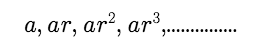is a GP with 'a' as the first term & 'r' as common ratio.

1.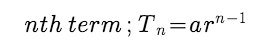2. Sum of first n terms ;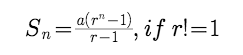3.Sum of infinite G.P ;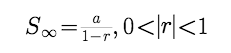PROPERTIES OF GP :

1. If each term of a G.P. be multiplied or divided by the some non-zero quantity, then the resulting sequence is also a G.P.

2. Three consecutive terms of a GP : a/r, a, ar ;

3. If a, b, c are in G.P. then b*b = ac.

4. If each term of a G.P. be raised to the same power, then resulting sequence is also a G.P.

If the terms of a given G.P. are chosen at regular intervals, then the new sequence is also a G.P.

Example :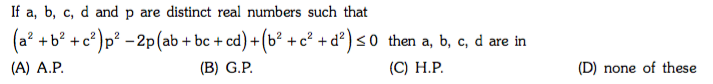Solution :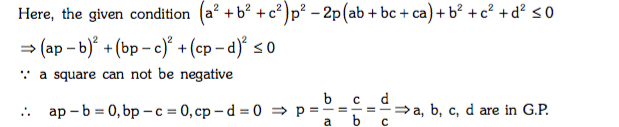Example :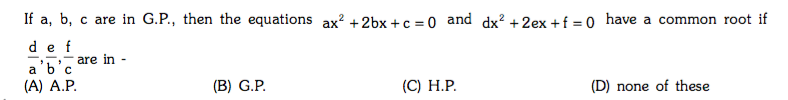Solution :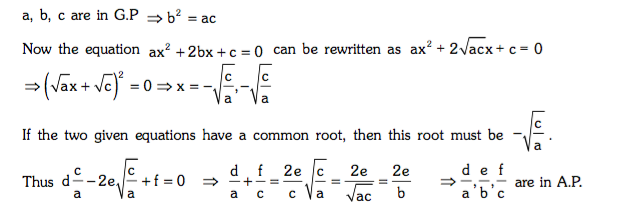Example :

A number consists of three digits which are in G.P. the sum of the right hand and left hand digits exceeds twice the middle digit by 1 and the sum of the left hand and middle digits is two third of the sum of the middle and right hand digits. Find the numbers.

Solution :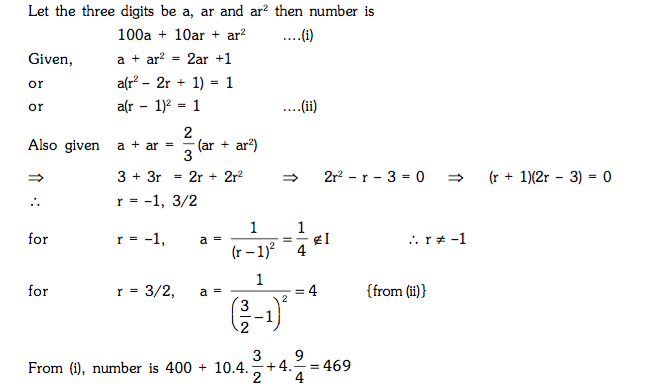Post By : Amit Rai 19 Apr, 2019 1914 views Maths